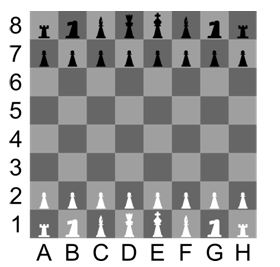Q:

# C++ program to determine the color of chess square

chess board is equally divided into 64 identical squares that are black and white alternately. Each square on the chessboard can be identified by the coordinates as 'A' to 'H' on the horizontal axis and '1' to '8' on the vertical axis as shown in the figure.Each square can be identified using the coordinate system specified above. For example, the square with coordinates G5 is colored BlackA6 is colored White and so on...

## Determine the color of a chess square board in C++

``````#include<iostream>
#include<cctype>
using namespace std;

int main()
{
char string, x;
cout << "Enter the coordinates of the square, \
\nthe first coordinate A to H and second coordinate 1 to 8: ";
cin.getline(string, 10);
x = string;
x = tolower(x);
string = x;
if (string == 'a' || string == 'c' || string == 'e' || string == 'g')
{
if (string == '1' || string == '3' || string == '5' || string == '7')
cout << "Black square";
else
cout << "White square";
}
else
{
if (string == '1' || string == '3' || string == '5' || string == '7')
cout << "white square";
else
cout << "Black square";
}

return 0;
}``````

Output

```First run:
Enter the coordinates of the square,
the first coordinate A to H and second coordinate 1 to 8: C5
Black square

Seccond run:
Enter the coordinates of the square,
the first coordinate A to H and second coordinate 1 to 8: F3
white square
```